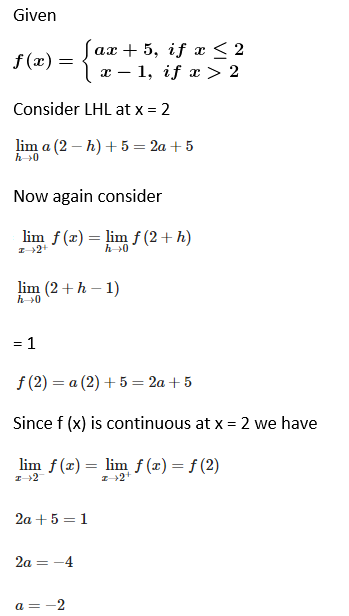# RD Sharma Solutions for Class 12 Maths Exercise 9.1 Chapter 9 Continuity

RD Sharma Solutions for Class 12 Maths Exercise 9.1 Chapter 9 Continuity are provided here. This exercise has problems which are solved on continuity of function by the subject experts at BYJU’S. Our team of faculty work with the aim of making Mathematics easier for students based on their grasping abilities. The students can gain a grip on the concepts covered in Maths Class 12 only by regular practice, and while solving, they can make use of the solution PDFs as reference materials. Students can easily download the RD Sharma Solutions for Class 12 Maths Exercise 9.1 of Chapter 9 from the below-provided links.

Let us have a look at the topics that are discussed in this exercise.

• The intuitive notion of continuity
• Continuity at a point
• Algebra of a continuous function
• Testing the continuity of a function at a point when the function has the same definition on both sides of the given point
• Testing the continuity of a function at a point when the function has different definitions on both sides of the given point
• Finding the values of a constant given in the definition of a function when it is continuous at an indicated point
• On the continuity of a composite function

## RD Sharma Solutions for Class 12 Maths Chapter 9 – Continuity Exercise 9.1:

### Access Another Exercise of RD Sharma Solutions For Class 12 Maths Chapter 9 – Continuity

Exercise 9.2 Solutions

### Access RD Sharma Solutions For Class 12 Maths Chapter 9 – Continuity Exercise 9.1

Exercise 9.1 Page No: 9.16

1. Test the continuity of the following function at the origin: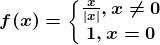Solution:

Given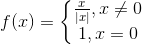Consider LHL at x = 0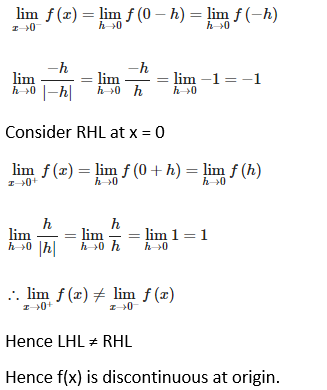2. A function f(x) is defined as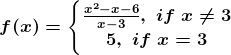Show that f(x) is continuous at x = 3.

Solution:

Given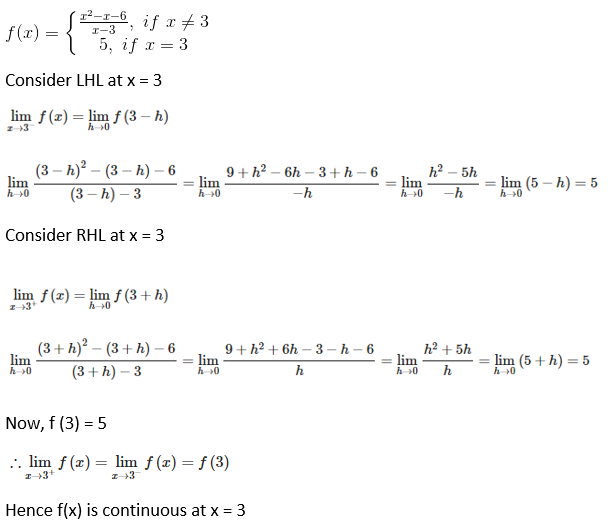3. A function f(x) is defined as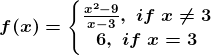Show that f(x) is continuous at x = 3.

Solution: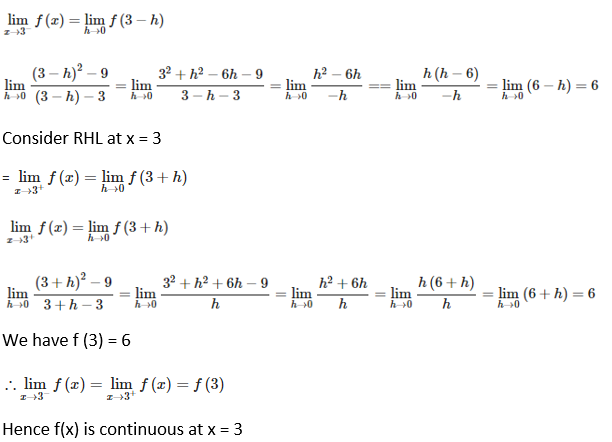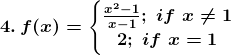Find whether f(x) is continuous at x = 1.

Solution: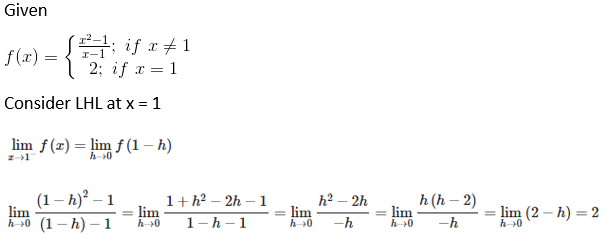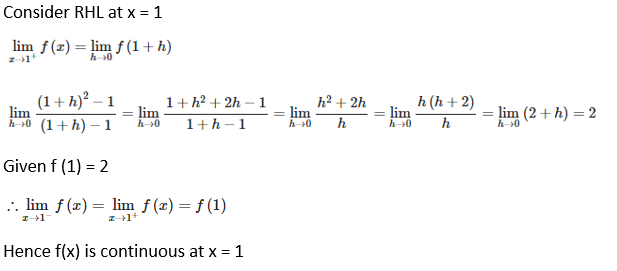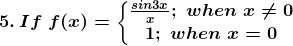Find whether f(x) is continuous at x = 0.

Solution: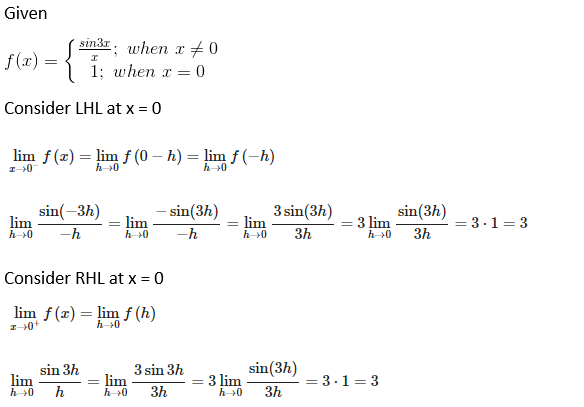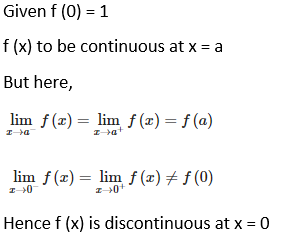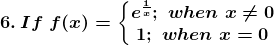Find whether f(x) is continuous at x = 0.

Solution:

Given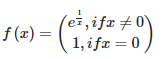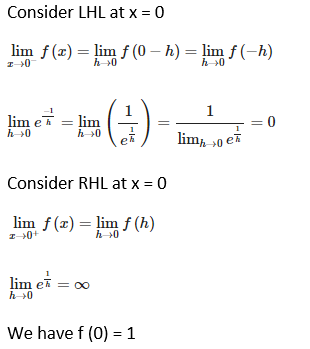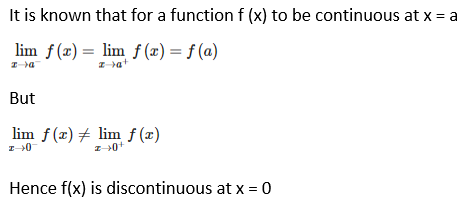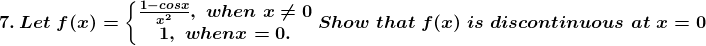Solution:

Given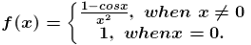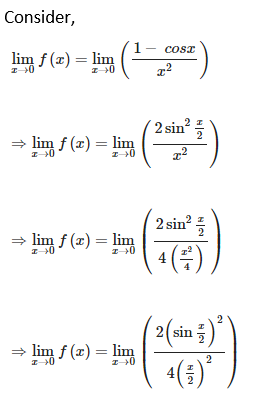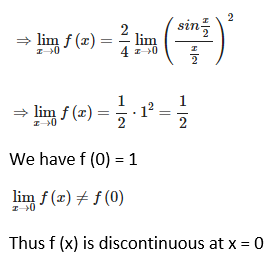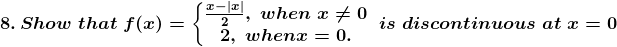Solution: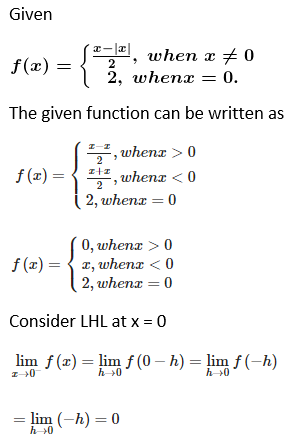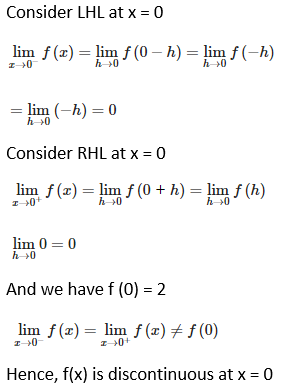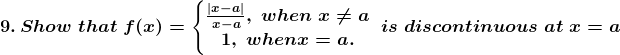Solution: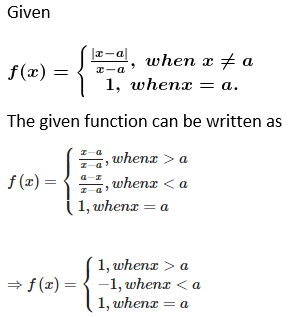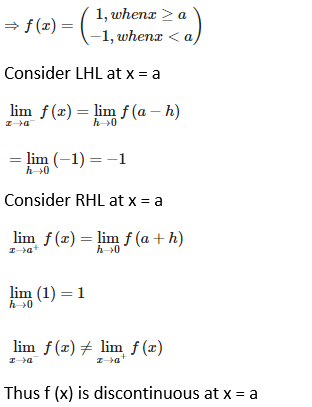10. Discuss the continuity of the following functions at the indicated point(s):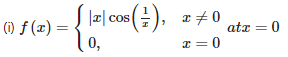Solution: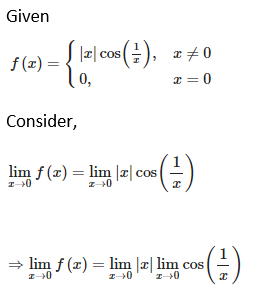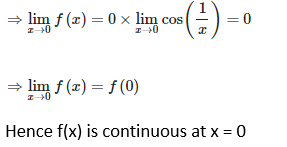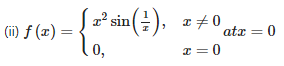Solution: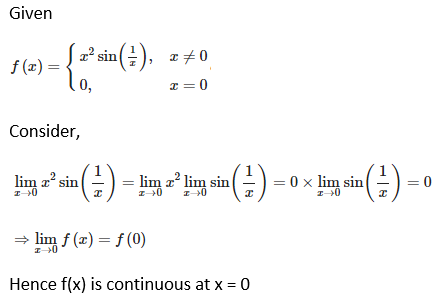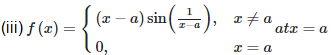Solution: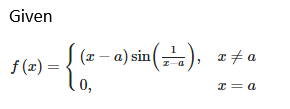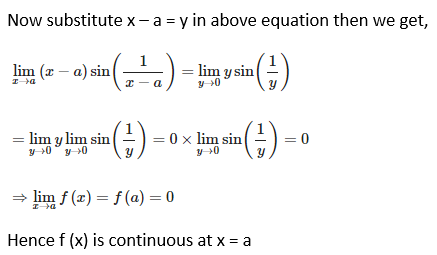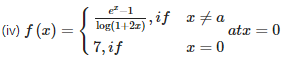Solution: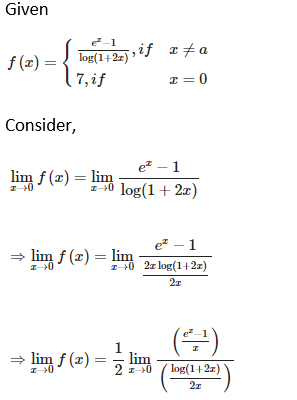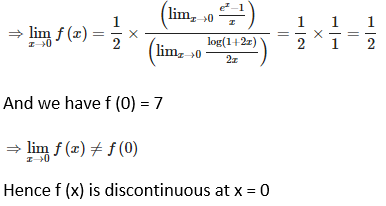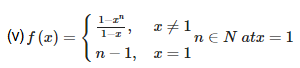Solution: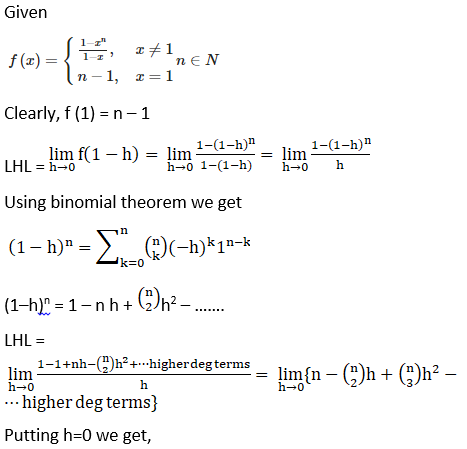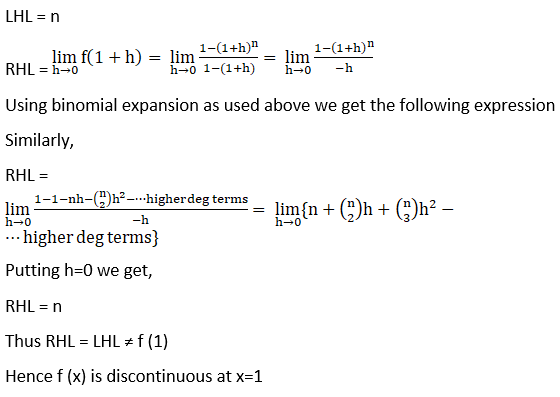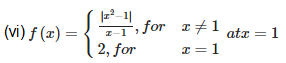Solution: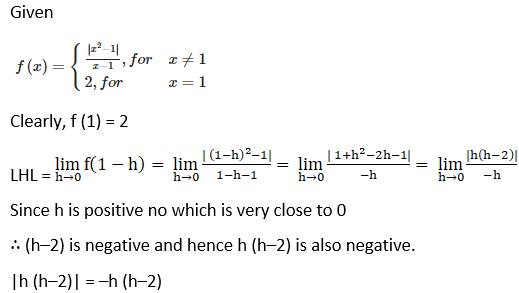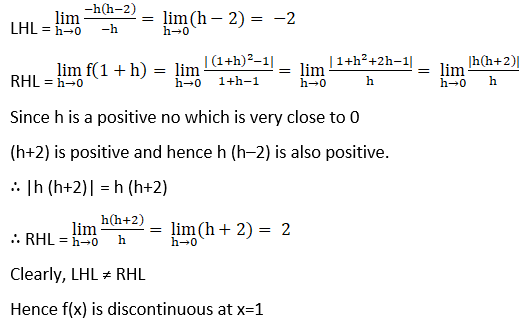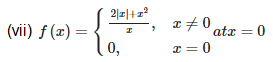Solution: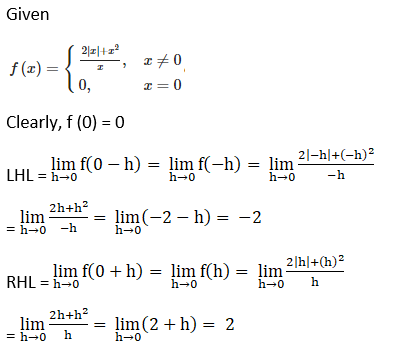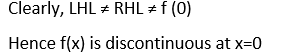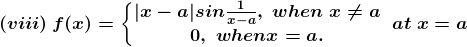Solution: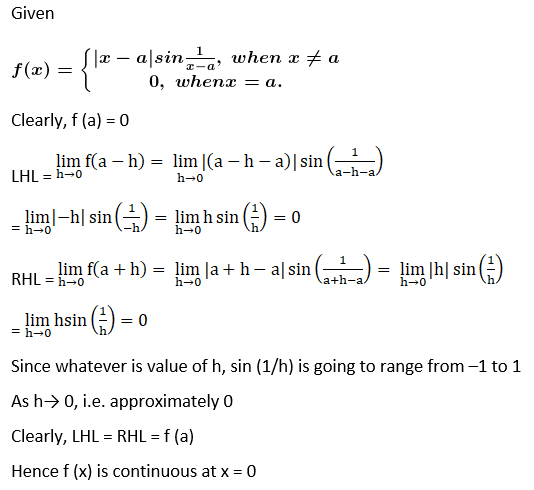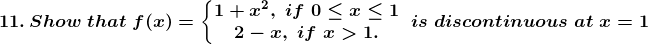Solution:

Given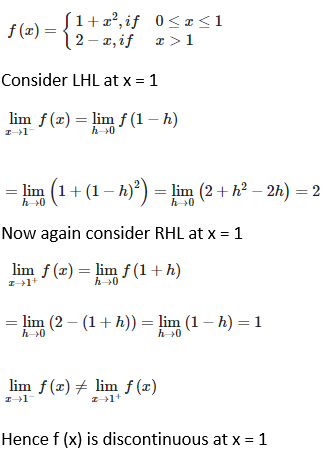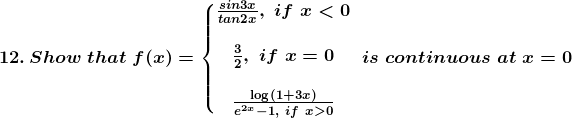Solution: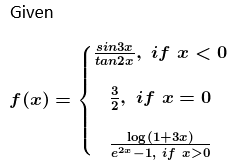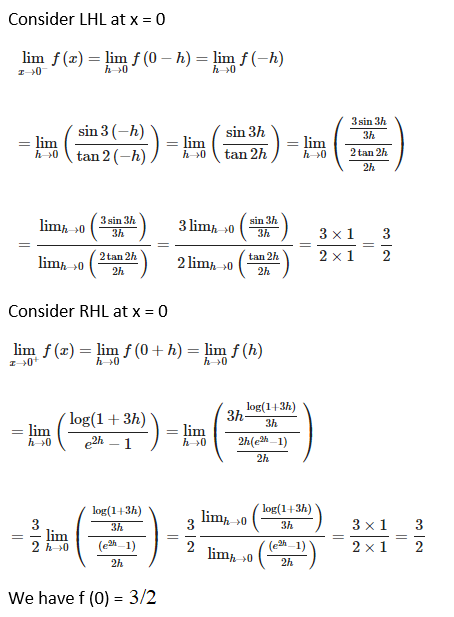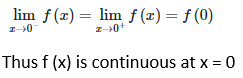13. Find the value of a for which the function f defined by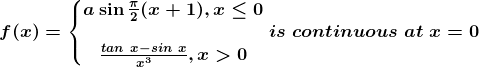Solution: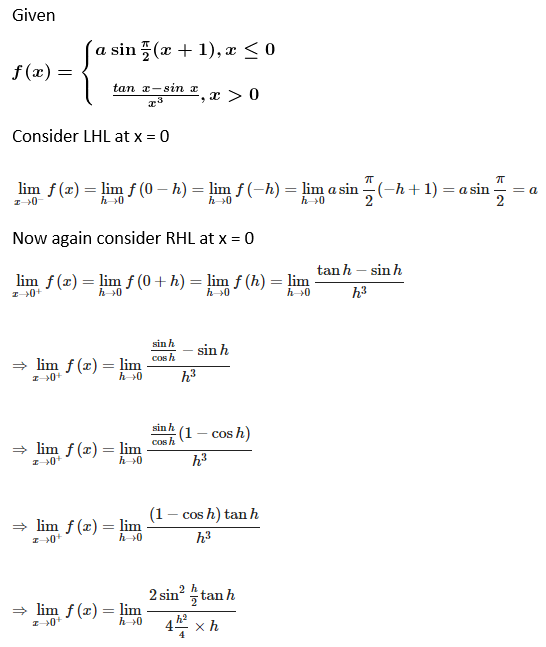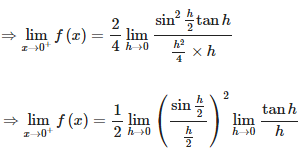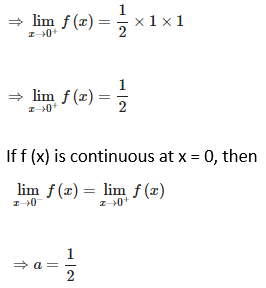14. Examine the continuity of the function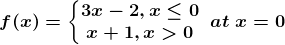Also sketch the graph of this function.

Solution: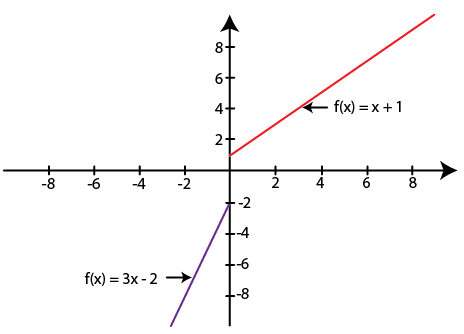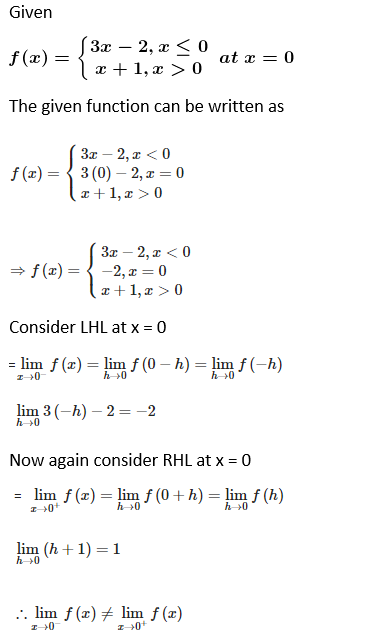Hence f (x) is discontinuous at x = 0

15. Discuss the continuity of the function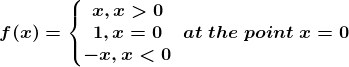Solution: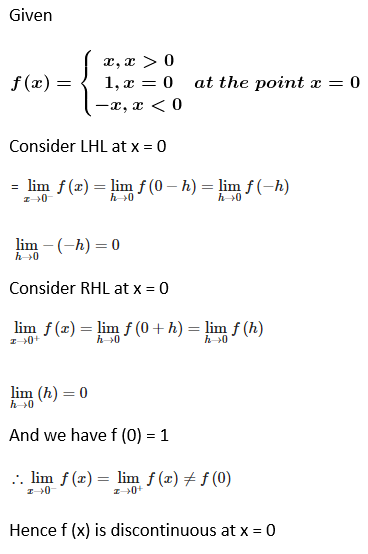16. Discuss the continuity of the function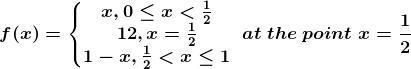Solution: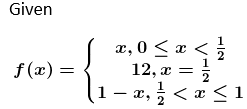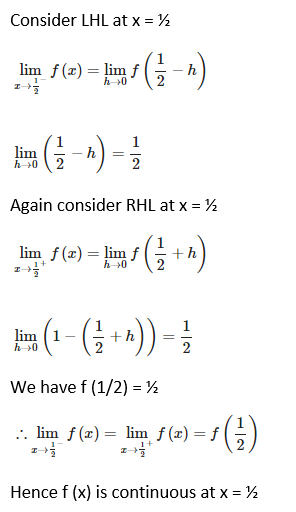17. Discuss the continuity of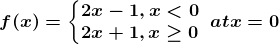Solution: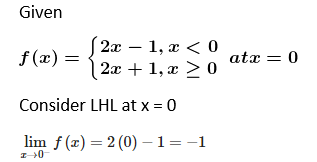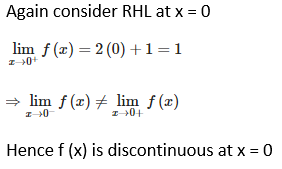18. For what value of k is the function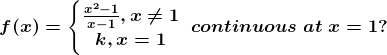Solution: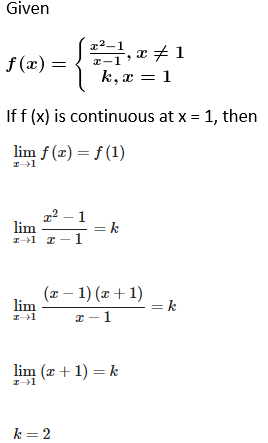19. Determine the value of the constant k so that the function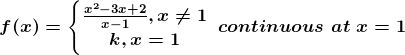Solution: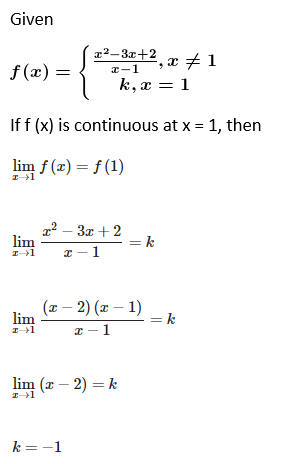20. For what value of k is the function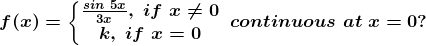Solution: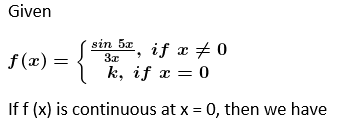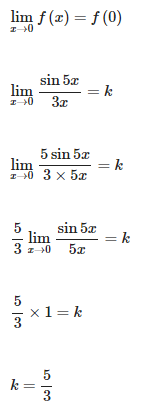21. Determine the value of the constant k so that the function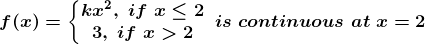Solution: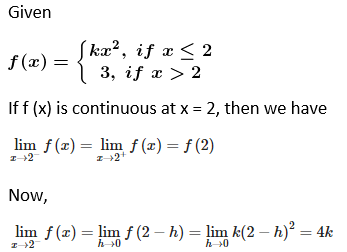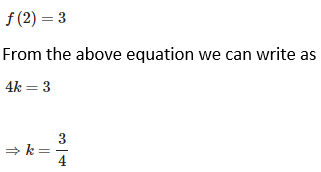22. Determine the value of the constant k so that the function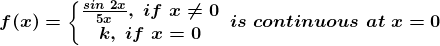Solution: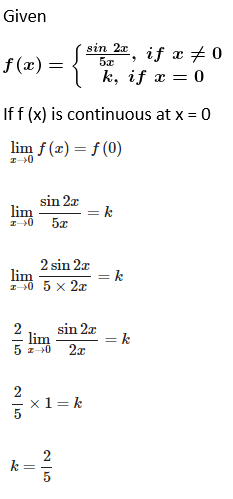23. Find the values of a so that the function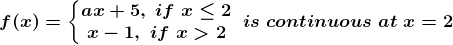Solution: